Search IntMath
Close

# IntMath Newsletter: Spherical spiral, calculators

By Murray Bourne, 20 May 2015

20 May 2015

1. How to find the length of a spherical spiral
2. Resource: EasyCalculation
3. More on Spirals
4. Math puzzles
5. Math movie: How to land on a comet
6. Final thought: The math narrative

## 1. How to find the length of a spherical spiral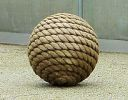How do we find the length of rope wound around a ball, with equidistant spirals? Here's the solution to an interesting reader's question. How to find the length of a spherical spiral

## 2. Resource: EasyCalculation

EasyCalculation.com has an amazing variety of online calculators, including not only the usual areas and angles, but also:

• Vector addition, angles and magnitude
• Chemistry (atomic mass, acids and bases)
• Finance
• Date and day
• Color converters (HEX, RGB and CSS)
• Mortgage and other finance related calculators
• Matrix operations

This free offering is a useful resource.

## 3. More on Spirals

I came across some interesting spiral examples while thinking about Anne's rope and sphere problem.

### (a) Escher's Art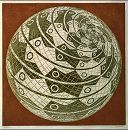Famous artist M. C. Escher used a spherical spiral in the piece Sphere Surface with Fish. The spiral in this image is like my first attempt at solving Anne's problem. See this, and other mathematical art by Escher here:

### (b) Music and spirals

Ever wondered why we use the notes we do in music? The 12 notes we have in an octave (7 white, 5 black on a piano) are no accident. They naturally occur as harmonics in nearly every note we hear.

For example, if we play the note A = 220 Hz on a piano, we also hear a mix of higher notes. We may not be aware of it, but those harmonics are what makes the difference in tone between a cello, a double bass, a bassoon and a pipe organ all playing the same note.

That A note on the piano also includes the following sounds, in various proportions:

• A one octave higher (440 Hz)
• E above that (659.3 Hz)
• A above that (880 Hz)
• C# above that (1108.7 Hz)

And so on, making up what is called the Harmonic Series. You may recognize the major chord A C# E A in these notes. (Different instruments when playing the same note include different proportions of these harmonics, hence producing different tones.)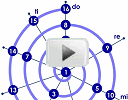This video illustrates this concept of harmonics contained within a musical note via the use of a spiral. Octave spiral with the first 16 elements of the harmonic series

### (c) Ribbon BodiesEscher also used spirals in his other artworks, including interpretations of clocks and human faces in the form of ribbons. Here's an image inspired by this approach: Wallpaper of Candice Swanepoel

## 4. Math puzzles

Correct answers with explanation were given by Sachin and Charles. It was great to see that Sachin made use of the mathematics entry possibilities in his answer.

### New math puzzle

Just after Anne's mail came in about the length of a spherical spiral (article above), I came across the following problem, which can also be done in at least 2 very different ways.

We have a cylnder with circumference 3 cm and height 12 cm. A piece of string is wound around the cylinder 4 times, as shown.

What is the length of the string?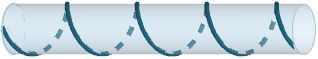## 5. Math movie - How to land on a comet (Fred Jansen)How do you align with a tiny rotating comet 600 million km away, travelling at 100,000 km/h, then land on it? This interesting TED talk has some insights into the mathematics behind the problem. How to Land on a Comet

## 6. Final thought - The math narrative

I'm lucky to live in a country (Singapore) where the prime minister is a math major.

Lee Hsien Loong studied mathematics at Trinity College, University of Cambridge. He shares the Trinity alumni list with Isaac Newton, James Clark Maxwell, Srinivasa Ramanujan, Charles Babbage, and Niels Bohr.

Lee did so well in his studies that he was named "senior wrangler", which means he was the top mathematics undergraduate, once regarded as "the greatest intellectual achievement attainable in Britain."

He recently posted his code to solve sudoku puzzles on Facebook, earning responses like "cool", "awesome" and "amazing".

When your leaders regard STEM subjects as vitally important to a country's future, it's not surprising the resources and encouragement follow. It's also not surprising how well the country does in international mathematics competitions. The consistent narrative in the country has emphasized the importance of science, mathematics and engineering.

I think it's tragic that so many world leaders are almost "anti-science", and doing very little about the environmental and other serious challenges we're facing.

Other notable persons with a mathematics background are:

• Corazon Aquino (former President of the Philippines)
• Alberto Fujimori (former President of Peru)
• Florence Nightingale (applied statistics to public health; invented the pie-chart)
• Lewis Carroll (author of Alice in Wonderland)

Until next time, enjoy whatever you learn.

1. cccccttttt says:

See you live in Singapore, a city that appears to have overcome 4 major cultures getting along.

I am a retired math teacher but now work seven days a week
as a private tutor.

Appreciate any links that would give an idea of the
demand for tutors in Singapore. They may have such a
good math program in schools there is no need.

Now live in Seattle also has blend of high tech and green. But it is also very dark and grey for 5 months a year....

(-:

ct

2. Murray says:

Hi

Considering Singapore citizen population of around 3.5 million, the annual figure of \$1 billion spent on tuition is quite remarkable.

http://www.straitstimes.com/singapore/education/tuition-industry-worth-over-1b-a-year

So yes, there certainly is a demand.

Singapore is rarely "dark and grey", but sometimes "darkish and brown" due to indiscriminate burning of rainforest in neighboring Indonesia.

3. Jaff Lawrence Asuiyi says:

Circumference C = 3cm
Height for each spiral h = (12/3)cm = 3cm
Length for each spiral L = hypotenuse
of right-angled triangle other sides C and h
L = sqrt(3^2 + 3^2)cm
L = 3sqrt(2)cm
Total length = 4L
= 12sqrt(2)cm
= 16.97cm

4. Thomas A Buckley says:

The string is 16.97 cm long.

Imagine the surface of the cylinder (and the string) cut, from a string end, along it's axis
and then flattened out.
Each of the 4 string turns will be a straight diagonal across a 3 cm x 3 cm square.
(That is 3 cm circumference by 12 cm length/4, the length of tube per turn.)
So the string length = 4 * Sqrt( 3x3 +3x3) = 4*Sqrt18 = 16.97 cm

PS. No back tick on iPad.

5. Tomas Garza says:

1. The circumference of the cylinder is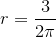.
2. One turn of a string around the cylinder takes 3 cm of string. If one end of the string is fixed and the other end is pulled uniformly away from its original position so as to reach a distance of 3 cm from the base of the cylinder, the string describes a helix.
3. The parametric equations of this helix are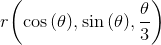.
4. I use the function ArcLength in Mathematica to calculate the length of the helix from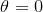to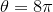, i.e., 4 complete turns. At this value the length of the cylinder is 12 cm. The length of the string is 12.65 cm.

6. Murray says:

@Thomas: There is actually a backtick on iPad, but it's troublesome to use (you need to hold down the apostrophe and then it appears as one of the choices.)

To make life easier, I've added the option to use q‍q as the delimiter as well. So your entry would look like:

q‍q = 4 sqrt( 3 xx 3 + 3 xx 3) = 4 sqrt18 = 16.97 q‍q,

giving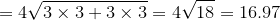7. Murray says:

@Jaff: Using the math entry system (see below), your solution could look like:

Circumference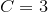cm

Height for each spiral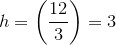cm

Length for each spiral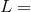hypotenuse

of right-angled triangle other sides C and h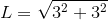cm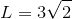cm

Total length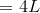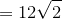cm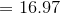cm

8. Zhuqi says:

1. we put the cylinder into a rectangle;the height is 12
2.we can divide the height into 4 equal parts.each one is 3
3. put the segment between the rectangle, is 3 sqrt2
4. there are 4 strings
4*3 sqrt2=12*sqrt2

9. Kaleigh Keley says:

I use This calculator to calculate the length of the helix from theta = 0 to theta = 8 pi, i.e., 4 complete turns. At this value the length of the cylinder is 12 cm. The length of the string is 12.65 cm.

### Comment Preview

HTML: You can use simple tags like <b>, <a href="...">, etc.

To enter math, you can can either:

1. Use simple calculator-like input in the following format (surround your math in backticks, or qq on tablet or phone):
a^2 = sqrt(b^2 + c^2)
(See more on ASCIIMath syntax); or
2. Use simple LaTeX in the following format. Surround your math with $$ and $$.
$$\int g dx = \sqrt{\frac{a}{b}}$$
(This is standard simple LaTeX.)

NOTE: You can mix both types of math entry in your comment.